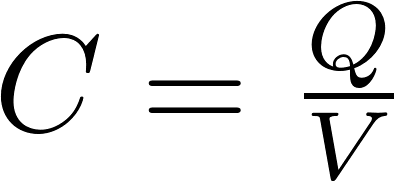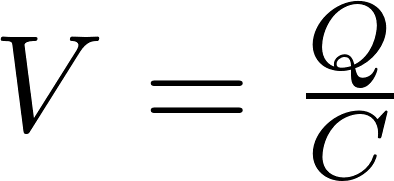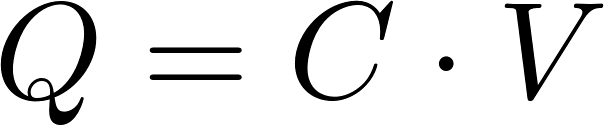# Capacitance calculator

Capacitance describes the ability to store electric charge. The unit for capacitance is farad (F), which is defined as one coulomb per volt (1 C/V). The capacitance (C) is equal to the charge (Q) divided by the voltage (V).

### Formulas

The capacitance, charge, and voltage can be calculated with one of the following formulas:C is the symbol for capacitance and is measured in farad (F).
V is the symbol for voltage and is measured in volt (V).
Q is the symbol for charge and is measured in coulomb (C).

### Calculator

Enter two values to calculate the third.